# Modelling

Living organisms and biological processes are usually complex to understand and their behavior hard to predict, therefore mathematical models help in simplifying the phenomena in question. In fact, these models, through their logical and scientific simplifications, help us predict the behaviours and through this, they help to define the experiments or even more, the project’s finalities.

Synthetic biology is based on a design test learn virtuous circle. We designed a genetic tool to enable in vivo directed evolution in microalgae, more particularly in Chlamydomonas reinhardtii. Our aim is to create a mutant library of the targeted gene, large enough to permit the directed evolution. We designed a genetic tool fully modular. The whole system is split into basic parts stored in Level 0 plasmids of the MoClo kit. This allows to change every element one by one or by combinatorial assembly. Our system is made to be improved through systematic assembly of different regulatory elements or retrotransposon parts. Selecting the construction by experimentally testing the synthetic transposons for every change is a very tedious and exhausting work. Moreover, getting a real estimation of the library’s size for every variation of the system is quite challenging. In fact, we would need an in silico model to find the most important and predictable bottleneck.

## Why do we need a model and influence of the model on the whole project

#### Initial thoughts

Initially, when we started working on ourin vivo directed evolution, one of the first questions was : “How do we know when to stop the induction of the retrotransposon to have the biggest library for a given sequence length in the shortest time with the smallest culture volume ?”. The next question was : what are the more important parameters that will have an influence on the library size? ”What are the parameters affecting the most the size of the library ?

Afterwards, we realized that the model can do much more than answer these questions. Modeling has a huge impact on our project. If we manage to have a reliable model, we can create a web tool helpful for in vivo directed evolution-users to choose the best different “MoClo parts” to assemble synthetic retrotransposons that would fit their applications.

The first step will be to make a first run with the CARGO and a standard Reverse-Transcriptase (RT). This run will allow users to test the activity of their construction and collect simple data that will help them to refine their construction. It will help scientists to find an answer to those questions (not exhaustive):

• Should I use a stronger promoter to induce the transposons?
• Should I use a different Reverse-Transcriptase?
• Should I use different LTRs/integrases?
• Is the CARGO sequence too big?
• Can I use more than one gene as CARGO?

The models need to use simple data that can easily be collected during the first retrotransposons experiments like the number of cells alive during RT induction, the number of RT inserted inside the genomic DNA, the transcriptomic level of the mRNA …

#### How modeling impacts our project

At the beginning, we were thinking of modelisation as a tool to get insight on how can we improve our retrotransposon. Our first modeling strategy needed experimental parameters than are tedious to obtain with complicated experiments and a lot of sequencing data.

We came up with a new strategy. Our second model which is more simple and robust : we could automate all the project because modeling was the perfect tool to screen the library size made by the retrotransposons directly from a 96-well microplate.

When we understood the usefulness of a model that could answer the previous questions, we came up with a new idea that will combine MoClo assembly, automation and modeling analysis.

MoClo assembly allows the quick and easy generation of a small library composed of a synthetic retrotransposon with differents parts. These assemblies can easily be generated by automation with the help of an Opentron OT-2 (protocols available for MoClo cloning). Once the synthetic transposons constructs are complete, they will be transformed in Chlamydomonas. By automated or manual colony picking, every clone will be cultured in a 96-well plate.

The next step was to calibrate the model. This will be done by automation with induction of the retrotransposons in 96-well microplates. Simple parameters will be monitored:

- Growth curve of Chlamydomonas while being induced. This will be used to calculate the half-life of the strain while induced. In other words, it measures the aggressivity of the retrotransposons (mobile elements can insert in essential genes etc…).
Number of retrotransposons inserted at the end of the induction. For our first test, we will consider that the number of inserted retrotransposons is linear correlated with time of induction.

Once these parameters are collected, they will be used by our model to determine the best synthetic retrotransposon construction for a given CARGO. It will also estimate the best induction time and optimal parameters (if they are tested in calibration experiments, for example induction temperature has a huge impact in our experiments).

This modeling study will provide preliminary results, before the generation of an in vivo library at scale (hundred milliliters of cultures or even liters) with optimized parameters.

Opentron OT-2 can be use for every step of this process. Most of the protocols are already online and it is easy to develop with Python the ones that will be specific to our iGEM project.

## First strategy

Our first approach was a mechanistic and dynamic model based on differential equations. The goal was to describe and integrate the individual parameters one by one at the molecular level (gene, RNAs, proteins etc...). We wanted to model what will happen in a single cell and then multiply by the number of cells implied to get the size of the library(Figure 2 ).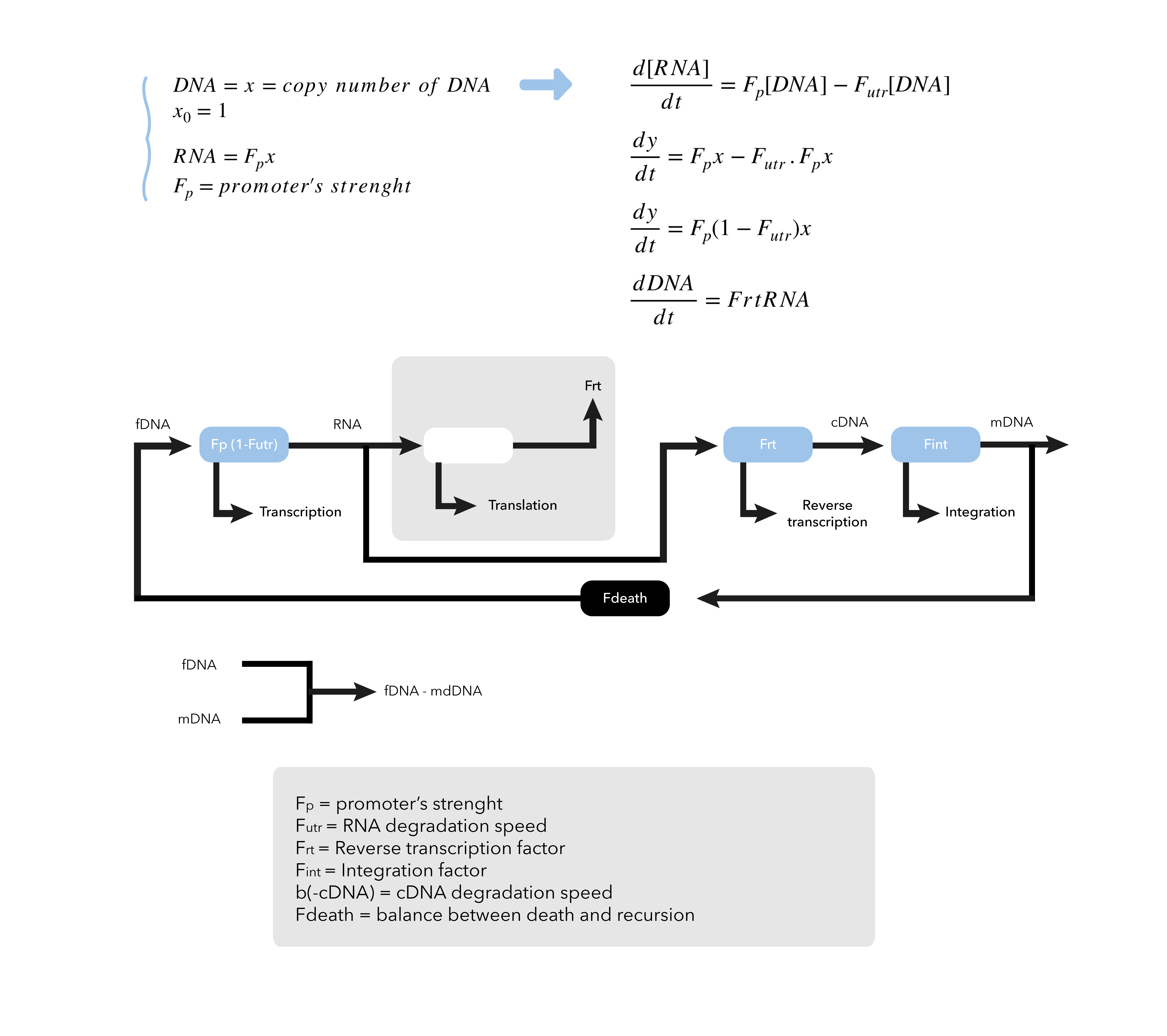Figure 2 Mechanistic and dynamic model with differential equations of our first approche

Here is an overview of the different parameters we wanted to integrate:

While the RT was still in construction, we could not use real parameters to calibrate our model. We started looking into the literature to find some data to run the model for a first trial. So, we wanted to perform a mathematical modeling of our system in order to predict the different factors involved.

While this model can be very efficient, a lot of experiments are needed to calibrate it properly. For example:

• Fp: The promoter of the retrotransposon is pNIT and based on previous reports, we estimated its force of transcription which helped us in calculating an approximate frequency of transcription of the construct.
• Futr: The number of transcribed RNAs is closely monitored in eukaryotes as the RNAs are usually degraded after exiting the nucleus. However, there are different conditions that lead to their degradations before they get translated or reverse- transcribed. In fact, several events can lead to the early degradation of the RNAs: the splicing of the introns can induce the total degradation of the RNA, mutated RNAs are eliminated as part of the quality control process and finally the “constitutive” degradation of RNA occurs regularly in an eukaryotic cell (Houseley and Tollervey, 2009). qPCR can be used to determine the quantity of mRNA over the time.
• Frt: Reverse Transcriptase (RT) that has a high error rate leading to random mutations in the resulting copy of the transcript.
• Fint: Number of retrotransposons integrated after induction. This depends of the aggressivity of the retrotransposons and should vary within the various types of retrotransposons. qPCR can be used to count the number of copies of the retrotransposon.
• b(-cDNA): After being spliced, the resulting RNA is treated by the Reverse Transcriptase that is encoded by the POL gene to generate a complementary DNA (cDNA). It has been reported that the cDNA in human and mouse cells are usually digested by a protein called TREX1 (Kawane et al., 2014). Since we are working with Chlamydomonas reinhardtii which is an eukaryotic cell, we assumed that the cDNA produced in our system would also be digested. In fact, this process would prevent its integration into the genome and therefore it stops the process of directed evolution. This step is a turning point in our system.
• Fdeath: cDNA is supposed to integrate into the genome with the help of integrase which is encoded by the pol gene of the retrotransposon. According to previous studies in plants, an LTR-retrotransposon found in plants, named tobacco Tnt1 elements known for its high transposition, integrates mostly into or next to gene-coding sequences and that these integrations are usually not detrimental for the host (Le et al., 2007). But there are still chances that the integration will lead to the death of the cell. This parameter can be monitored with the growth curve of the cells while being induced.

After some literature research, we do not pursued with this model as too much work was needed, with the risk of getting results that we can not easily confront with the real events. In fact, even if the parameters we wanted to calibrate could be obtained experimentally, it needed too much work and optimization that would not fit into the tight schedule of the iGEM competition. Plus, the differents experiments to get the data needed are not easy to adapt to automation. Moreover, every variable and parameters can be the source of variability making the strategy too complex and not reliable for our needs.

## A more robust and easy model to calibrate

We decided to switch strategy for the model and developed a simplest one that way more robust with an analytical solution. Only two experimental parameters are needed: i) a growth curve while induction of RT and ii) the number of integrated RT. This model will allow us to determine the best induction time to generate the largest library.

• Growth curve and half-life of yeast cells : 96 h. We make the hypothesis that the half-life of microalgae will be much longer than that of yeast. We choose to describe the population of microalgae with an exponential model survival. We think the process of survival after the integration is without memory. That means that if a cell has already 3 integrated retrotransposons, the chance of kill it after a fourth integration has the same probability if the cell has zero retrotransposons or 10.
• Number of integrated retrotransposons per cell : We used 1 transposon per cell (0.02 for yeast). The microalgae’s genome has much more transposable elements than yeast (up to 30%). For example in Volvox carteri there are 80 copies of Osser in the genome. In yeast, there are only 5 copy of Ty16. For the linear function that describe the number of retrotransposons over time. We made this postulate because it simply the model for the first run. This function can be change and refine if needed. We will need more insight and experimental data to find a function that describe perfectly the reality.
• CARGO sequence length : We use a cargo of 1000 base pairs.

Let $$\frac{1}{\lambda}$$ be the average survival time of a cell, we suggest an exponential model of survival: $x(t) = e^{-t\lambda}$ To obtain $$\lambda$$ from $$t_{\frac{1}{2}}$$ the half-life: $\frac{1}{2} = e^{-t_{\frac{1}{2}}\lambda}$ $-\log{2} = -t_{\frac{1}{2}}\lambda$ $\boxed{\lambda = \frac{\log{2}}{t_{\frac{1}{2}}}}$ So for $$t_{\frac{1}{2}}=24\mathrm{h}$$: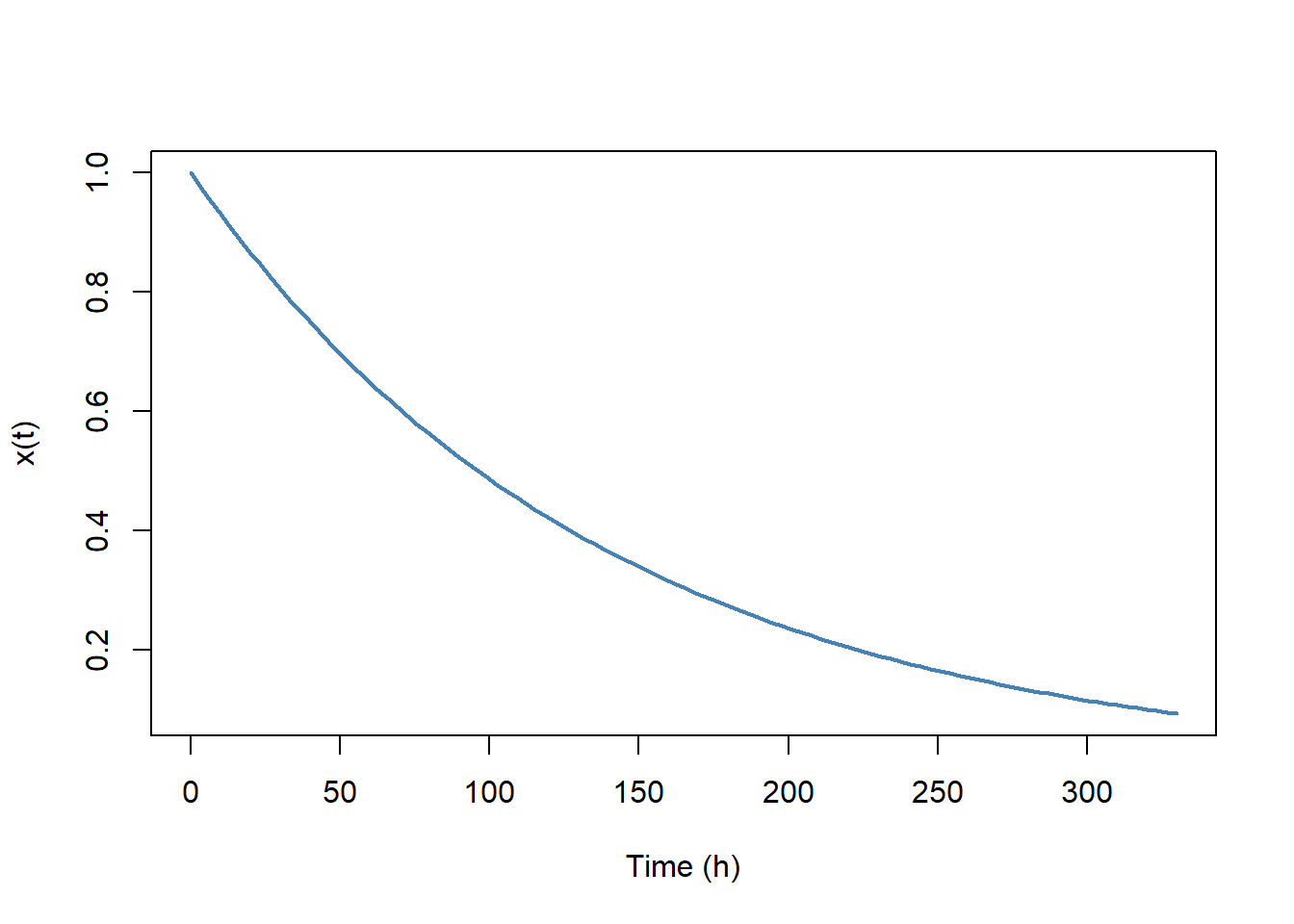Let us suppose the number $$y$$ of transposons per cell is a linear function of time, and that there is for example the number of transposons $$y\left(t_{\frac{1}{2}}\right) = y_{t_{\frac{1}{2}}}$$ then: $y(t) = t\frac{y_{t_{\frac{1}{2}}}}{t_{\frac{1}{2}}}$ So we have for $$y_{t_{\frac{1}{2}}} = 1$$: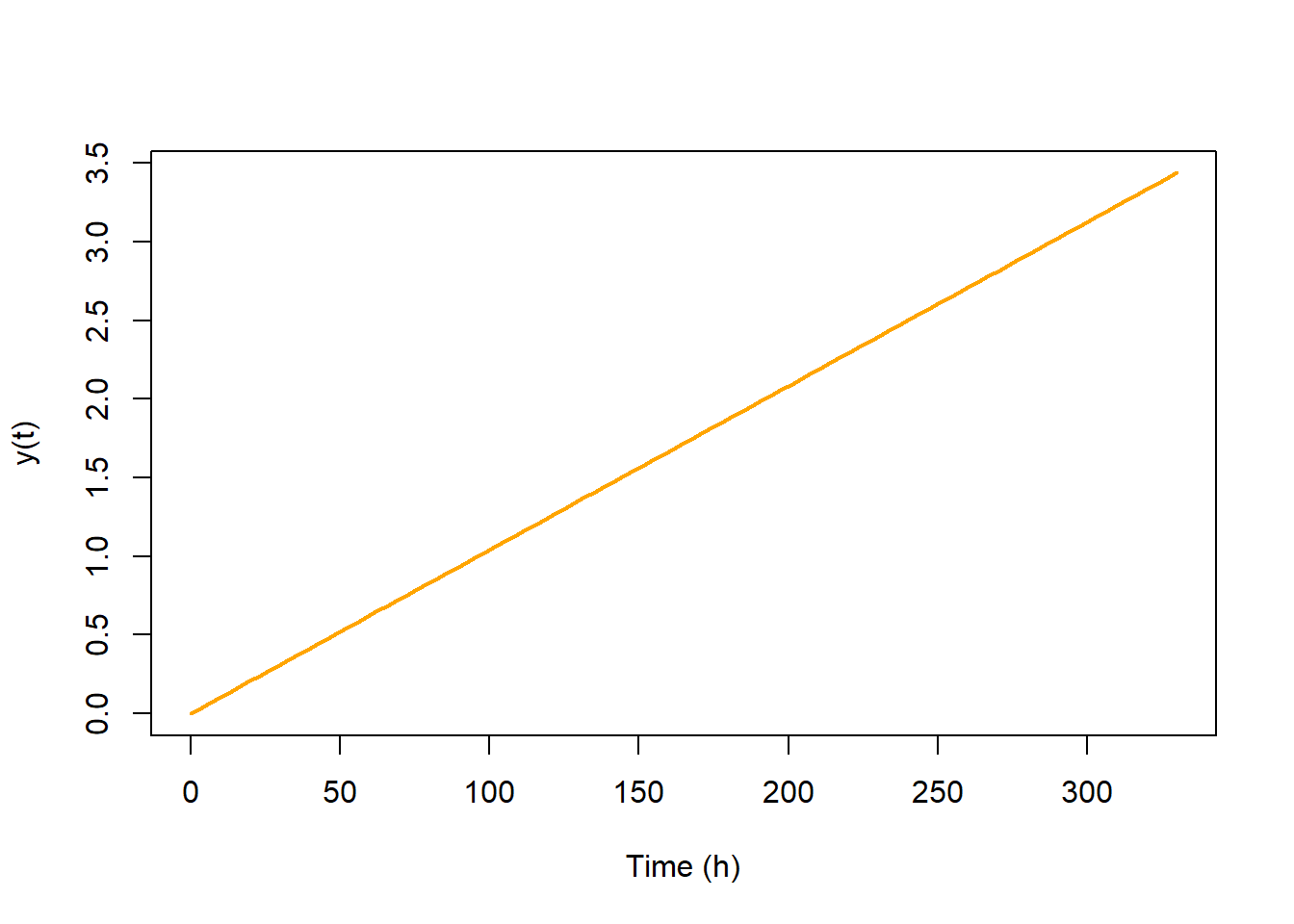Finally we propose $$z(t)$$ the number of transposons in the culture as being the product of the number of cells by the number of transposons per cell:: $z(t) = x(t)y(t)$ And therefore: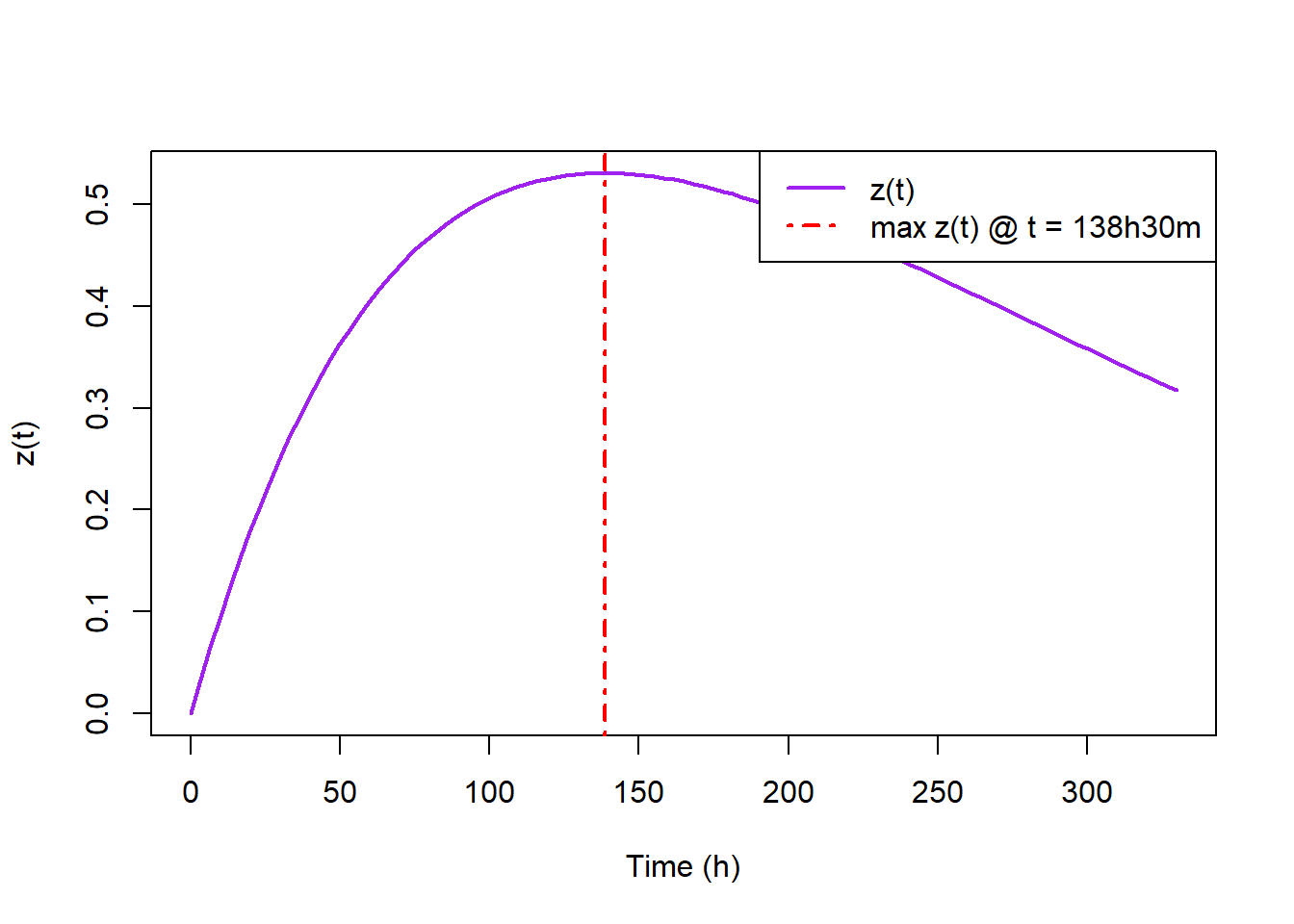Once the number of transposons is obtained, the question of the number of unique alleles generated L comes. Consider the Coupon collector’s problem: a collector looking to have all the coupons of a particular series however at the time of purchase the coupon’s number is unknown; the question is how much does take to have the complete collection? One can show that the solution is a sum of random variables according to a geometrical law (cf.Wikipedia). In the Coupon collector’s problem the draw probabilities are uniform, but in reality mutations are not at all uniform, we will figure out how to overcome this difficulty.

Let us proceed by steps:

1. Let us suppose that each nucleotide in the DNA sequence of interest of length $$N$$ has a uniform probability $$p$$ to mutate into one of the three other possible nucleotides. In this case for a given transposon, the number of mutated bases $$K$$ is a random variable following a binomial law : $$K \sim B(N, p)$$ and so of expectation $$\mathbb{E}(K) = Np$$

2. Let us separate all of the possible alleles in $$N+1$$ subsets $$A_k\quad (k \in0..N)$$ each containing the $$a_k$$ alleles with the same number $$k$$ of mutated bases. Let us determine the value of the $$a_k = \#A_k$$ (cardinal of $$a_k$$ meaning the number of elements in $$A_k$$). For any element of $$A_k$$ we select $$k$$ modified nucleotides among $$N$$, each of which can have one of 3 new values possible. So we have : $a_k = {N \choose k}3^k \quad \left(= \frac{N!3^k}{(N-k)!k!}\right)$

3. Each mutation of a nucleotide having a uniform probability, then within one $$A_k$$ all alleles are equiprobable. We get back to the Coupon collector’s problem if one considers only a subset. However the solution to the Coupon collector’s problem gives us the number of necessary transposons $$T_{L_k}$$ on average to obtain a certain number of unique alleles $$L_k$$, and not the inverse that interests us, that is the number of alleles $$L$$ depending on the number of transposons $$T$$.

$\mathbb{E}(T_{L_k}) = a_k\sum_{j=0}^{L_k-1}\frac{1}{a_k-j}$

1. To obtain the solution of the inverse problem, the simplest way is to proceed by framing: if $$\mathbb{E}(T_{L_k}) \le T \le \mathbb{E}(T_{L_k+1})$$ then $$L_k \le L \le L_k+1$$

2. We know how many integrated transposons we have, from the first point we know their distribution by number of mutations, and from the second and third points we can find the number of unique alleles per number of mutations, so we have enough information to solve the whole problem, meaning to determine the number of unique alleles that we can expect.

To illustrate, let us consider at the optimal time: $\begin{cases} N = 1000\\ p = \frac{1}{1500}\\ \mathrm{cells} = 8,000,000,000 \end{cases}$

##     K       L.k
## 1   0         1
## 2   1      3000
## 3   2   4495500
## 4   3 106240523
## 5   4  17877883
## 6   5   2375773
## 7   6    262830
## 8   7     24897
## 9   8      2061
## 10  9       151
## 11 10        10
##  "Total : 131282791"

## Test of the model with data published by Crook and al. (2016) on Saccharomyces cerevisae

Since we could not use data provided by our system in Chlamydomonas, we decided to use the data of the original paper of Crook et al to confront our model with the reality5.

Then we used parameters determined from the original paper of Crook et al 2016 to confront our model with experimental results5:

• Growth curve and half-life of yeast cells : 48 h. We match this value so the maximum inducing time determined by our model is roughly the same as the inducing time used in the paper (3 days)
• Number of integrated retrotransposons per cell : We used 0.02 transposons per cell.
• CARGO sequence length : We use a cargo of 1000 base pairs.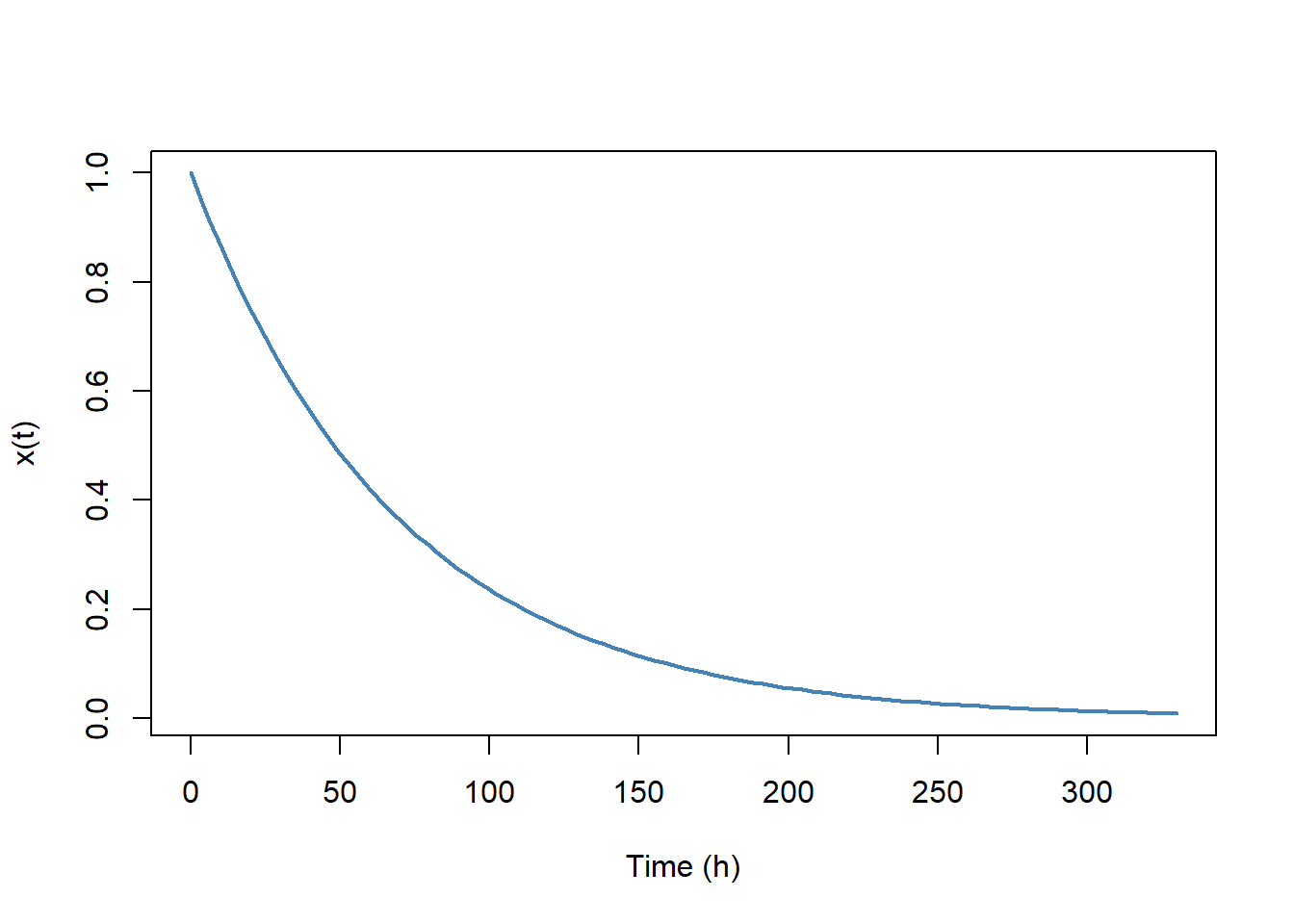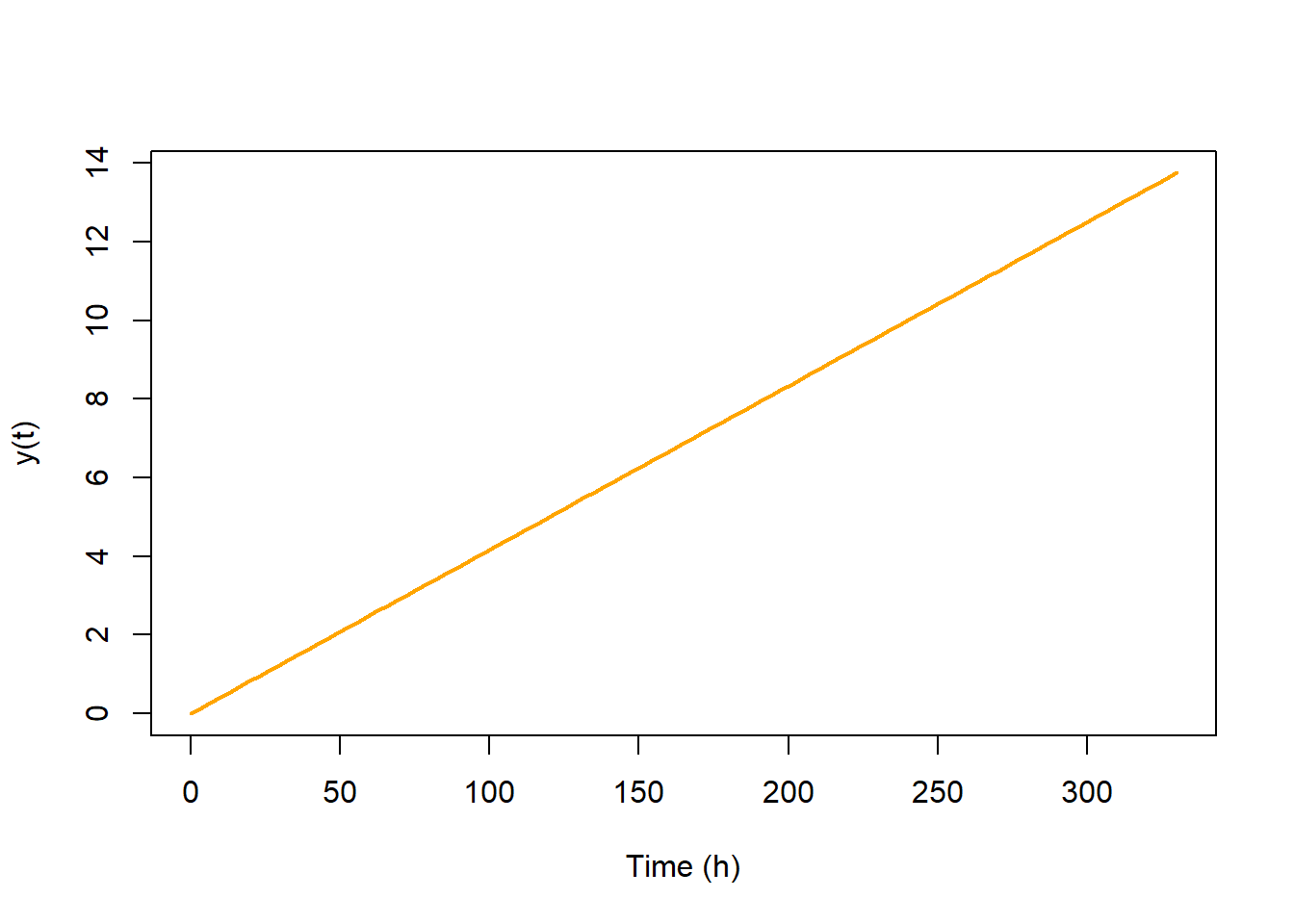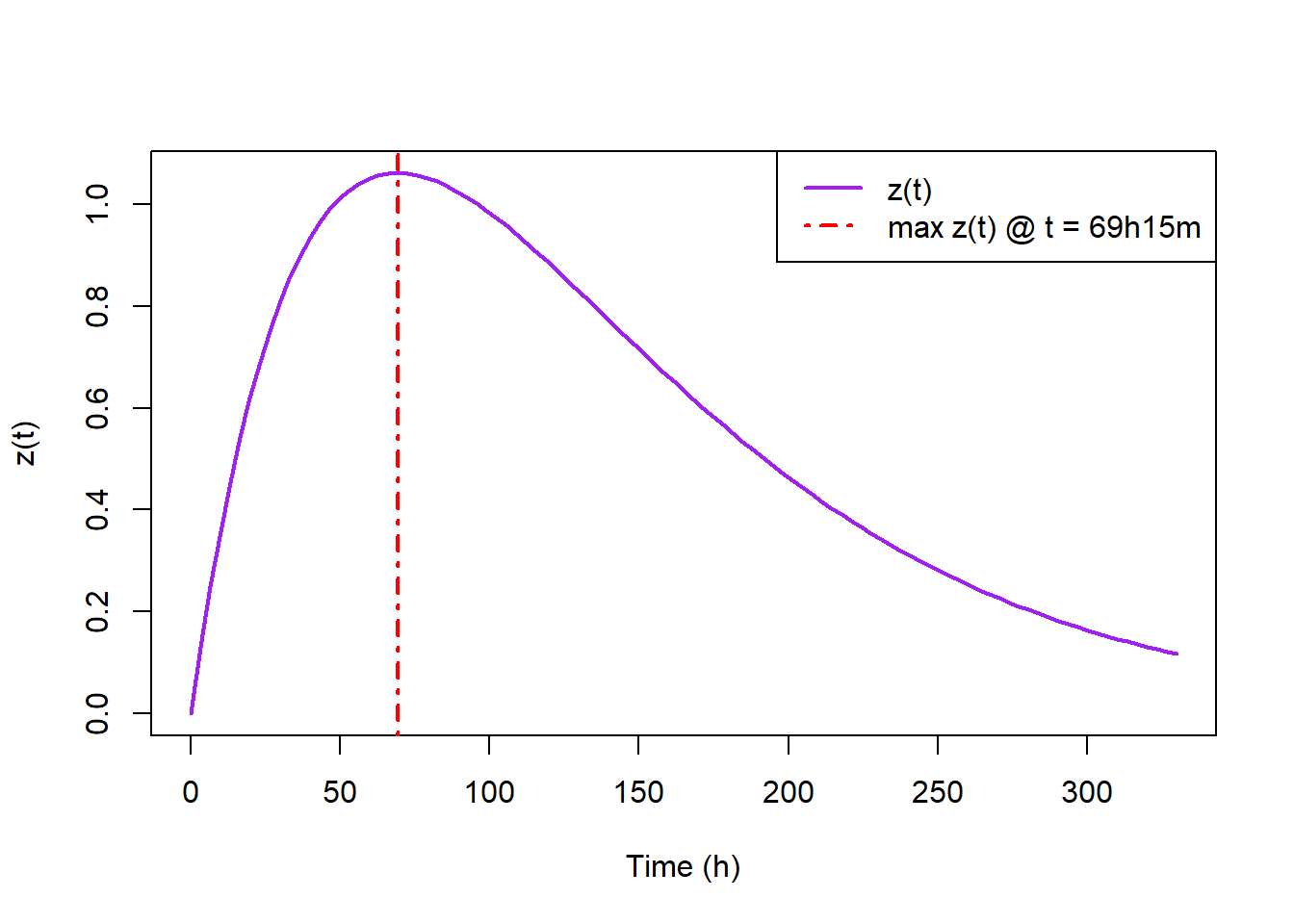$\begin{cases} N = 1000\\ p = \frac{1}{1500}\\ \mathrm{cells} = 8,000,000,000 \end{cases}$

##     K       L.k
## 1   0         1
## 2   1      3000
## 3   2   4495500
## 4   3 209965272
## 5   4  35755671
## 6   5   4751546
## 7   6    525660
## 8   7     49795
## 9   8      4123
## 10  9       303
## 11 10        20
## 12 11         1
##  "Total : 255551054"

Our model finds a library size of : 2.5 million mutants for 8 billion cells. When we translate for 10 billion cells, 3.1 million of mutants are generated. Crook et al 2016, estimated 2.7 millions with the use of the PEDEL software. In order to use this software, they isolated and sequenced hundreds of colonies to count how many times 3 RT mutants (one with 1 mutation, 1 with 2 mutations and 1 with 3 mutations) can be isolated. This is an expensive and tremendous work.

Some work is still needed to fully validate and refine our model with differents conditions. Moreover, we will need to test the whole system once we have finished with the construction of our first synthetic retrotransposon. Nevertheless, those preliminary results are promising because they are matching real experiments from Crook et al work on S. cerevisiae and retrotransposon Ty1.

To conclude, our model could be used to characterize the activity of synthetic retrotransposons in a high-through pipeline. We can automate the construction of different retrotransposons because we design it to be fully modular with the use of a MoClo kit. Then by using qPCR experiments and OD measurements in 96-well microplates we can screen for the best construction. This can only be done using the GoldenGate cloning system along with our model.